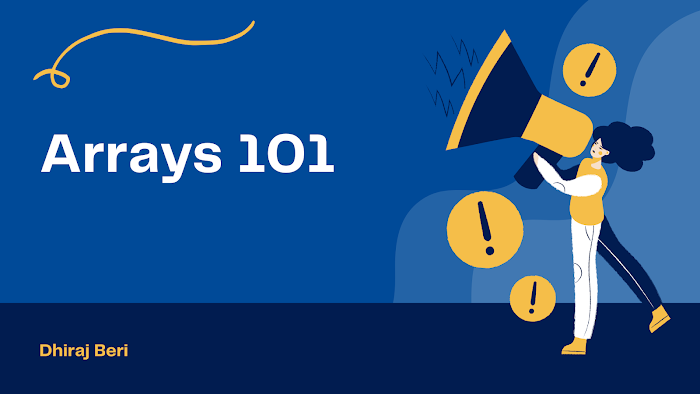# Finding a Pair of Elements with sum K

a+b = k

`#include <stdio.h>int main(){    int a[] = {5, 8, 3, 9, 6, 2, 10, 7, -1, 4};    int i, j;    int n = 10;    int k;    scanf("%d", &k);    for (i = 0; i < n - 1; i++)    {        for (j = i + 1; j < n; j++)        {            if (a[i] + a[j] == k)            {                printf("%d+%d=%d\n", a[i], a[j], k);            }        }    }    return 0;}`

Using hashing
`#include <stdio.h>int main(){    int a[] = {5, 8, 3, 9, 6, 2, 10, 7, 1, 4};    int h = {0, 0, 0, 0, 0, 0, 0, 0, 0, 0};    int i, j;    int n = 10;    int k;    scanf("%d", &k);    for (i = 0; i < n; i++)    {        if (h[k - a[i]] != 0)        {            printf("%d + %d = %d\n", a[i], k - a[i], k);        }        h[a[i]]++;    }    return 0;}`

Sorted array
`#include <stdio.h>int main(){    int a[] = {1, 3, 5, 7, 9};    int i, j, k, n;    n = 5;    k = 10;    i = 0;    j = n - 1;    while (i < j)    {        if (a[i] + a[j] == k)        {            printf("%d + %d = %d\n", a[i], a[j], k);            i++;            j++;        }        else if (a[i] + a[j] < k)        {            i++;        }        else        {            j--;        }    }    return 0;}`

Do you have any other optimal solution for this problem? Try to solve this by yourself and don't focus on the optimal solution if you are a beginner. Just try to solve it by yourself. Good Luck ;)